CATEGORIES:

# The Intersection of a Plane and a straight Line

Suppose given a plane Ax+By+Сz+D=0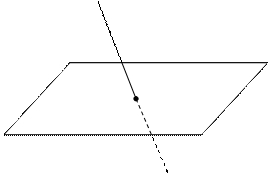and a straight line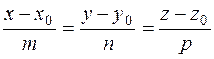in space.

If the straight line and the plane do not intersect, then the parameter t1 does not exist. Suppose, that the line is parallel to the plane; then the vectors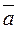and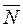are perpendicular, i.e., or (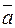·)=0, and Am+Bn+Cp=0. This means that the denominator vanishes.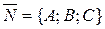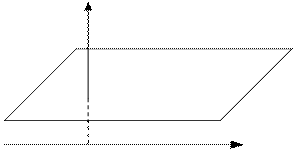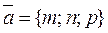Thus, the parallelism condition for a straight line and a plane is

Am+Bn+Cp=0.

Suppose that the line is perpendicular to the plane; then the vectorsandare collinear, i.e., their coordinates are proportional: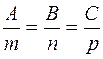.

This is the perpendicularity condition for a straight line and a plane.

Example. Write the equation of a straight line perpendicular to the plane 2x-3y+4z+11=0 and passing through the intersection point of this plane with the line.

Let us reduce the canonical equation to the parametric form: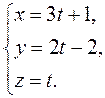Substituting these expressions for the variables x,y, and z into the equation of the plane

6t+26t+6+4t+11=0.

Thus, 4t=–19,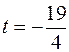, and the intersection point of the line with the plane has the coordinates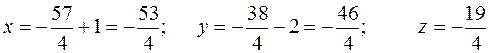.

The normal vector to the plane is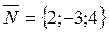; since the straight line is perpendicular to the plane, it follows that its direction vector is collinear to the normal vector, i.e.,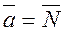. Thus, the required straight line is determined by the equations.

The angle between a plane and a straight line. Suppose that a plane in space is determined by its general equation

Ax+By+Сz+D=0,

and a straight line is determined by its canonical equations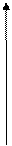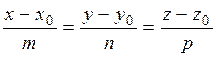.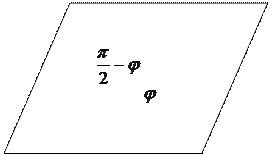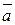Take the acute angle between the right line and its projection on the plane for the anglebetween the straight line and the plane.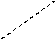Using the scalar product of vectorsand the general scheme, we determine the angle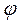from the angle between the direction vector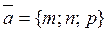and the normal vector;.

By the reduction formula,whence.

Definition. A directed interval (or an ordered pair of points) is called a vector.В

АDefinition. A vector with coinciding endpoints is called the null vector.

Definition. The distance between the head and tail of a vector is called the length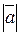, or absolute value of this vector. It is denoted byor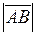.

Definition. Vectors are collinear if they lie on the same straight line or on parallel lines.

A B C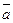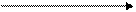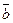are collinear vectors,

А1 В1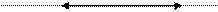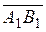,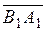are collinear vectors.

Definition. Vectors are coplanar if they lie in the same plane or in parallel planes.

Definition. Two vectors are said to be equal if they are collinear and have the same direction and length.

Date: 2015-01-02; view: 1596

 <== previous page | next page ==> STRAIGHT LINES IN SPACE | Linear Operations on Vectors
doclecture.net - lectures - 2014-2023 year. Copyright infringement or personal data (0.017 sec.)